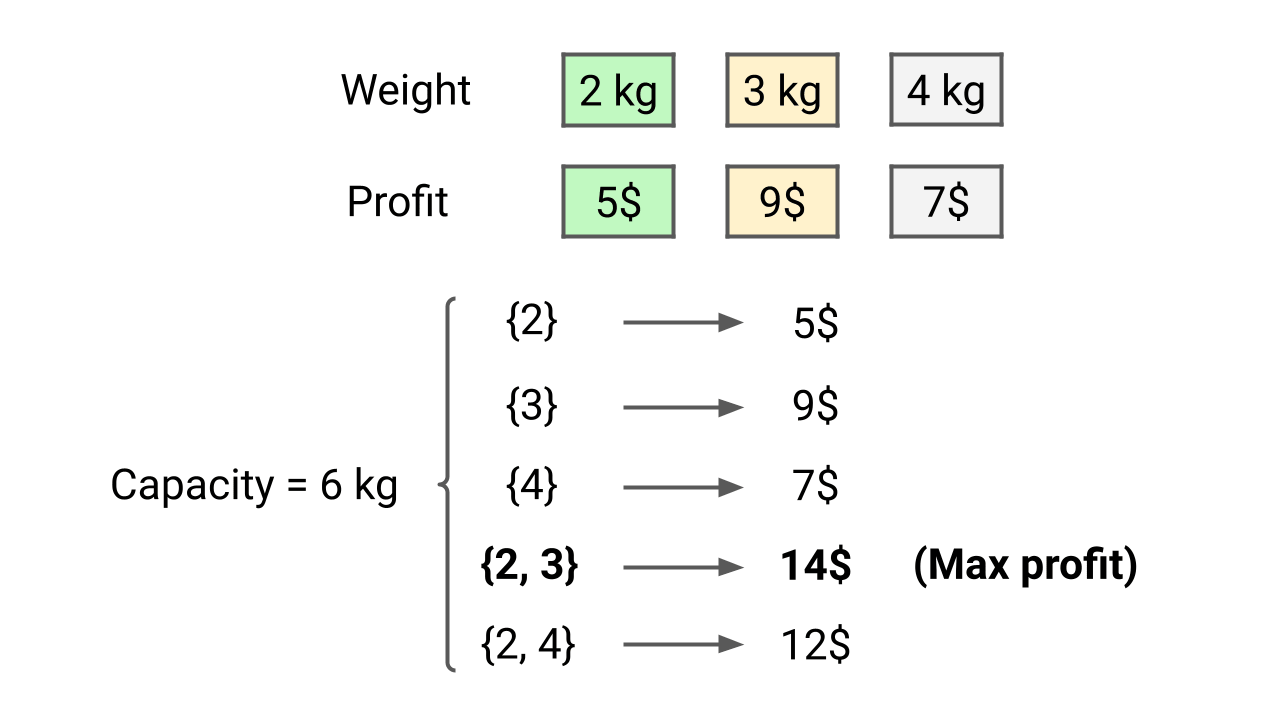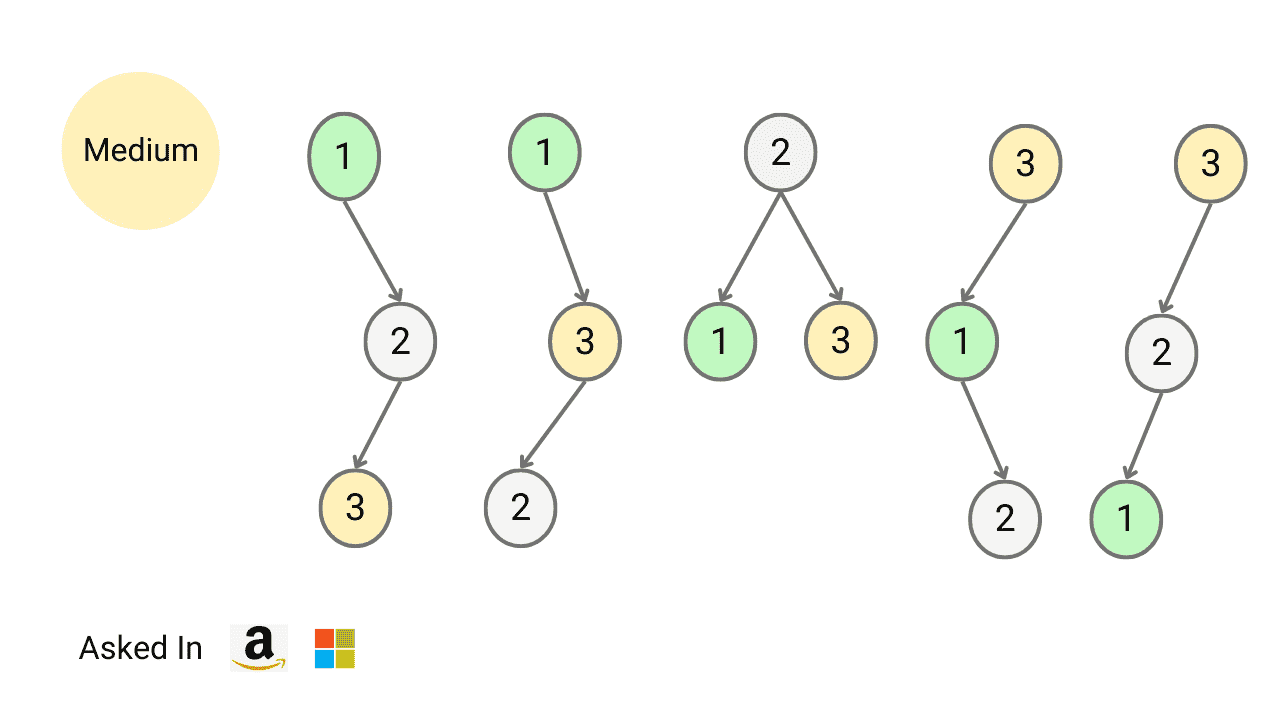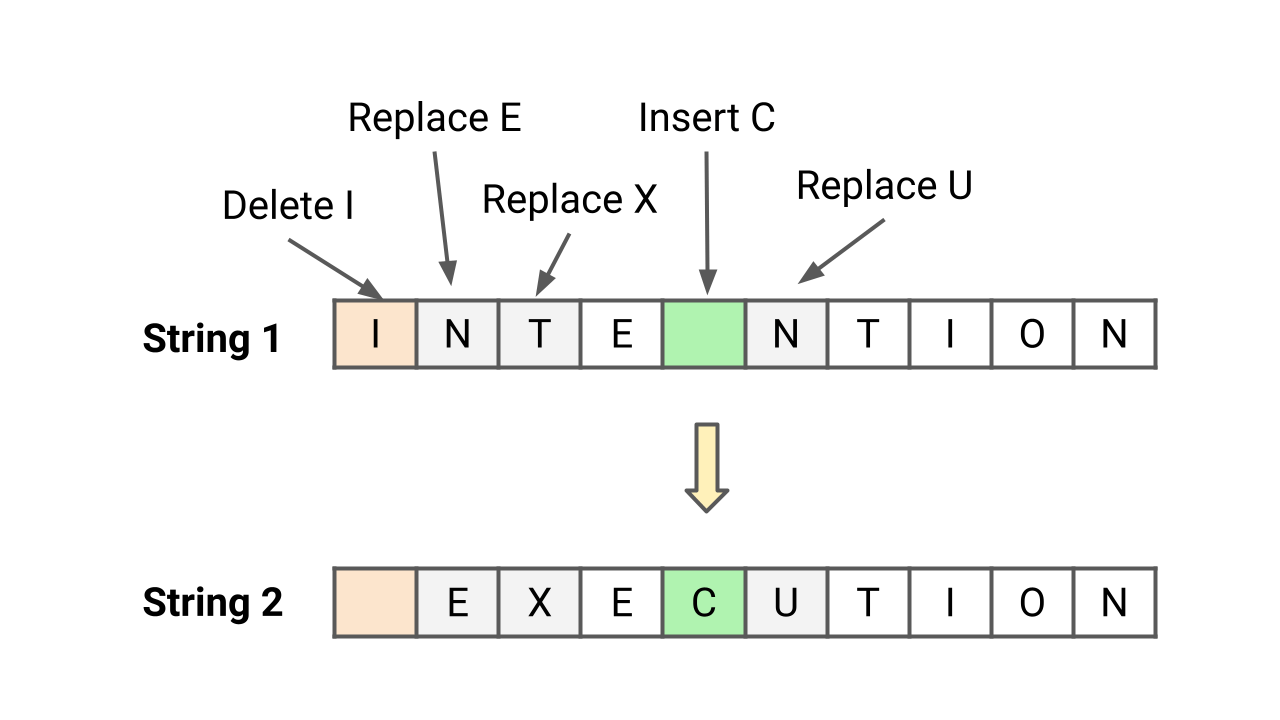# dynamic-programmingMinimum Number of Jumps to Reach End

An array of non-negative integers is given and the aim is to reach the last index in the minimum number of jumps. You are initially positioned at the first index of the array and each element in the array represents your maximum jump length at that position. Note: This is an excellent problem to learn problem solving using dynamic programming.Given an array of n elements, write a program to find the maximum subarray sum. A subarray of array X[] is a contiguous segment from X[i] to X[j], where 0 <= i <= j <= n-1. Note: Max subarray sum is an excellent problem to learn problem-solving using the divide and conquer approach, dynamic programming, and single loop (kadane's algorithm).Divide and Conquer vs Dynamic Programming

Divide and conquer and dynamic programming differ in terms of implementation, analysis, and the nature of the subproblems. Divide and conquer divides the problem into independent subproblems, solves them recursively, and combines them to get the solution. In dynamic programming, problems are divided into dependent subproblems and solved in an order to get the solution.0-1 Knapsack Problem

We are given n items, where each item has a weight and a profit associated with it. We are also given a knapsack with a capacity of C (the knapsack can hold a maximum weight of C). Write a program to determine the maximum profit that can be obtained by selecting a subset of items without exceeding the knapsack's capacity.Longest Common Subsequence

Given two strings X[] and Y[] of sizes m and n, design an algorithm to find the length of the longest common subsequence (LCS). There can be many possible common subsequences of two strings, but we need to return the common subsequence of the longest length. Note that this is an excellent problem to learn dynamic programming.Count Total Number of Unique Binary Search Trees with n Keys

Write a program to find the number of structurally unique binary search trees (BSTs) that have exactly n nodes, where each node has a unique integer key ranging from 1 to n. In other words, we need to determine the count of all possible BSTs that can be formed using n distinct keys.Edit Distance (Levenshtein Distance) Problem

Write a program to find the minimum number of operations required to convert string X to string Y. You have the following three operations permitted on a string: 1) Insert a character 2) Delete a character 3) Replace a character. The edit distance between two strings is the minimum number of operations (insertions, deletions, or substitutions of characters) required to transform one string into the other.Climbing Stairs Problem

There is a staircase with n steps, and you can climb either 1 or 2 steps at a time. Write a program to count and return the number of unique ways to climb the nth stair. The order of steps taken matters. Note: Climbing stairs is an excellent problem to learn the dynamic programming approach and the application of the Fibonacci series in problem-solving.Minimum Coin Change Problem

Suppose we want to make a change for a given value K of cents, and we have an infinite supply of each of coin[ ] = [C​1​​, C​2​​, …, C​m​​] valued coins. Write a program to find the minimum number of coins required to make the change. Note: This is an excellent counting problem to learn problem solving using dynamic programming approach.Introduction to Dynamic Programming in DSA

Dynamic Programming is a popular problem solving approach in data structures and algorithms, which solve problems by combining subproblem solutions like divide and conquer. But rather than solving the same sub-problem again, DP solves sub-problems once and stores the calculated value in extra memory to avoid the recomputation.Top-Down (Memoization) vs Bottom-up (Tabulation) in DP

Understanding differences between top down (memoization) and bottom up approach (tabulation) of dynamic programming will help us make critical decisions during problem-solving. One idea is common to both approaches: They use extra memory to store solutions to sub-problems, avoid recomputation and improve performance.Types of Problems Solved Using Dynamic Programming

There could be two popular categories of problems that can be solved using dynamic programming: 1) Optimization problem: Here we need to find an optimal solution (minimum, longest, shortest, etc.) from a large solution space 2) Counting problem: Here we need to count different ways to find all occurrences of a combinatorial pattern.